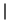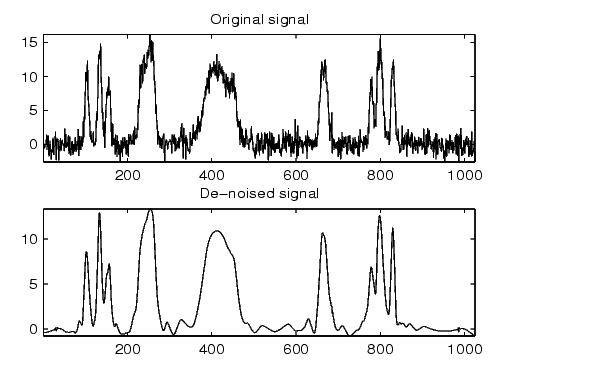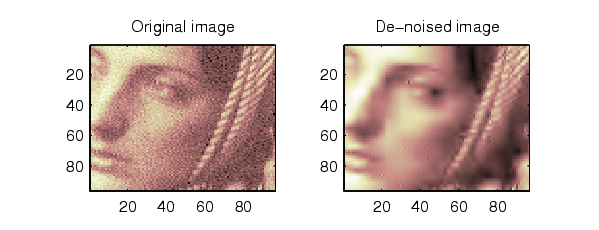Wavelet Toolboxwbmpen

Penalized threshold for wavelet 1-D or 2-D de-noising

Syntax

• ```THR = wbmpen(C,L,SIGMA,ALPHA)
```

Description

THR = wbmpen(C,L,SIGMA,ALPHA) returns global threshold THR for de-noising. THR is obtained by a wavelet coefficients selection rule using a penalization method provided by Birge-Massart.

[C,L] is the wavelet decomposition structure of the signal or image to be de-noised.

SIGMA is the standard deviation of the zero mean Gaussian white noise in de-noising model (see `wnoisest` for more information).

ALPHA is a tuning parameter for the penalty term. It must be a real number greater than 1. The sparsity of the wavelet representation of the de-noised signal or image grows with ALPHA. Typically ALPHA = 2.

THR minimizes the penalized criterion given by

let t* be the minimizer of

• ```crit(t) = -sum(c(k)^2,k<=t) + 2*SIGMA^2*t*(ALPHA + log(n/t))
```

where c(k) are the wavelet coefficients sorted in decreasing order of their absolute value and n is the number of coefficients; then THR =c(t*).

wbmpen(C,L,SIGMA,ALPHA,ARG) computes the global threshold and, in addition, plots three curves:

• 2*SIGMA^2*t*(ALPHA + log(n/t))
• sum(c(k)^2,k<=t)
• crit(t).

Examples

• ```% Example 1: Signal de-noising.

% Perform a wavelet decomposition of the signal
% at level 5 using sym6.
wname = 'sym6'; lev = 5;
[c,l] = wavedec(x,lev,wname);
% Estimate the noise standard deviation from the
% detail coefficients at level 1, using wnoisest.
sigma = wnoisest(c,l,1);

% Use wbmpen for selecting global threshold
% for signal de-noising, using the tuning parameter.
alpha = 2;
thr = wbmpen(c,l,sigma,alpha)
thr =

2.7681

% Use wdencmp for de-noising the signal using the above
% threshold with soft thresholding and approximation kept.
keepapp = 1;
xd = wdencmp('gbl',c,l,wname,lev,thr,'s',keepapp);

% Plot original and de-noised signals.
figure(1)
subplot(211), plot(x), title('Original signal')
subplot(212), plot(xd), title('De-noised signal')% Example 2: Image de-noising.
nbc = size(map,1);

% Perform a wavelet decomposition of the image
% at level 3 using coif2.
wname = 'coif2'; lev = 3;
[c,s] = wavedec2(X,lev,wname);

% Estimate the noise standard deviation from the
% detail coefficients at level 1.
det1 = detcoef2('compact',c,s,1);
sigma = median(abs(det1))/0.6745;

% Use wbmpen for selecting global threshold
% for image de-noising.
alpha = 1.2;
thr = wbmpen(c,l,sigma,alpha)

thr =

36.0621

% Use wdencmp for de-noising the image using the above
% thresholds with soft thresholding and approximation kept.
keepapp = 1;
xd = wdencmp('gbl',c,s,wname,lev,thr,'s',keepapp);

% Plot original and de-noised images.
figure(2)
colormap(pink(nbc));
subplot(221), image(wcodemat(X,nbc))
title('Original image')
subplot(222), image(wcodemat(xd,nbc))
title('De-noised image')```

```wden, wdencmp, wpbmpen, wpdencmp ```waverec2 wcodemat